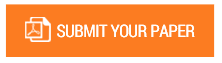Statistical Solution to Complex Markov Matrix Chains

Authors : Dr. Ismail Abbas

Volume/Issue : Volume 6 - 2021, Issue 3 - March

Scribd : https://bit.ly/2QkEdGZ

We divide this article into two parts. In part 1, we introduce a complex Markov matrix, propose and validate a statistical technique to its solution called the complex stationary eigenvector of Markov chains. We show that statistical techniques are more efficient and more precise than the classical algebraic method of solving a linear system of algebraic equations of the homogeneous Markov system. The statistical solution fails only when the Markov matrix is not invertible. In this case, the classic solution also fails. In part 2, we introduce a stochastic transition matrix B other than the Markov transition matrix. The transition matrix B can be real or complex as well as the Markov matrix. Likewise, we propose and validate a statistical solution to complex B-Matrix transition chains.nThe proposed B-Matrix (nxn) and its B-Matrix chains is valid for any 2D and 3D configuration for any arbitrary number of free nodes n. In addition, we extend the validity of the hypothesis principle applied for real B-Matrix chains to the case of complex B-Matrix chains: [For a positive symmetric physical matrix, the sum of their powers at the eigenvalues is equal to the eigenvalue of their sum of the series of powers of the matrix]. In the current article, we provide a numerical validation of this principle by comparing the eigenvalue of the sum of the series of powers of the matrix B with the sum of the series of infinite powers of the eigenvalues of the Bmatrix itsel

#### CALL FOR PAPERS

Paper Submission Last Date
31 - May - 2022